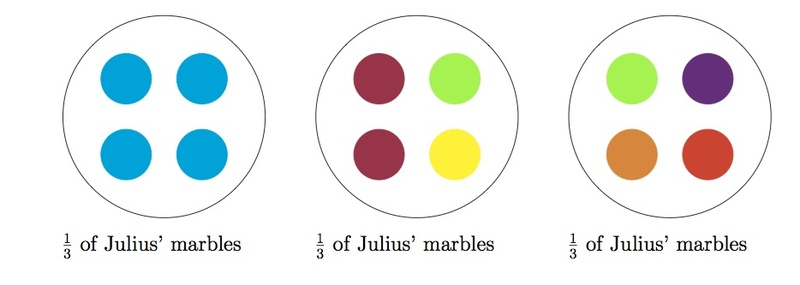# How many marbles?

Alignments to Content Standards: 5.NF.B.7.b 5.NF.B.7.c

Julius has 4 blue marbles. If one third of Julius' marbles are blue, how many marbles does Julius have? Draw a diagram and explain.

## IM Commentary

This task is intended to complement "5.NF How many servings of oatmeal?" and "7.RP Molly's run.'' All three tasks address the division problem $4 \div \frac{1}{3}$ but from different points of view. "How many servings of oatmeal'' presents a how many groups version of $4 \div \frac{1}{3}$ while "Molly's run" approaches this division problem from the point of view of rates. This task provides a how many in each group version of $4 \div \frac{1}{3}$.

To recall in this setting the difference between a how many groups version and a how many in each group version of the equation $$4 \div \frac{1}{3} = 12$$ notice that there are two different multiplication equations which can give rise to this division equation:

\begin{align} 12 \times \frac{1}{3} &= 4 \\ \frac{1}{3} \times 12 &= 4. \end{align}

In the first of these equations $12$ groups of $\frac{1}{3}$ make $4$ and so $12$ is the answer to how many thirds there are in $4$. In the second equation $\frac{1}{3}$ of a group of $12$ make $4$ and so $12$ is the answer to how much is in a group if one third of that group is $4$.

This task should be done together with the "How many servings of oatmeal" task with specific attention paid to the very different pictures representing the two situations. A good picture will determine which version of division, how many groups or how many in each group, is being modeled in a given situation.

This task is appropriate for assessment or for instruction.

## Solutions

Solution: 1

If one third of Julius' marbles are blue, this means that $\frac{2}{3}$ of his marbles are colors other than blue. This means that Julius has two times as many marbles of colors other than blue than he has blue marbles. Since Julius has $4$ blue marbles, this means that he has $8$ marbles of other colors and so he has $12$ marbles total.

Below is a picture representing Julius' marbles:Since $\frac{1}{3}$ of Julius' marbles are blue, we can also record this as a missing factor problem: $\frac13 \times$ ____ $= 4.$ This missing factor problem is equivalent to the division problem: $4 \div \frac13 =$ ____. In either case, the answer is 12 marbles, as we saw in the picture.

Solution: Using a tape diagramThe number of blue marbles is represented by 1 unit. We can find the total using the missing factor sentence:

$4 = \frac13 \times$ ____.

This missing factor sentence is equivalent to the division problem:

$4 \div \frac13 =$ ____.

As the diagram shows, this is equivalent to solving:

$4 \div \frac13 = 4 \times 3 = 12.$

Julius has 12 marbles.